# Dickman-de Bruijn function

(diff) ← Older revision | Latest revision (diff) | Newer revision → (diff)

## Definition

This function, called Dickman's function or the Dickman-de Bruijn function, is defined as the function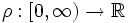satisfying the delay differential equation: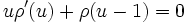subject to the initial condition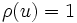for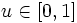. The function satisfies the following properties:

•for.
•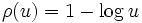for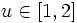.
•is (strictly) decreasing for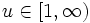, i.e.,for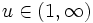.
•is once differentiable on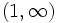. More generally, \$\rho[/itex] istimes differentiable everywhere except at the points.
•is infinitely differentiable except at integers.
• Failed to parse (syntax error):

.

## Related facts

It turns out that the density of numbers with no prime divisor greater than theroot is given by. Formally, consider, for any, the fraction of natural numbers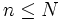such that all prime divisors ofare at most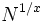. Then, as, this fraction tends to.